# Price Weather Derivatives

This example demonstrates a workflow for pricing weather derivatives based on historically observed temperature data.

The workflow for pricing call and put options for weather derivatives includes these steps:

The techniques used in this example are based on the approach described in Alaton .

### What Is a Weather Derivative?

A weather derivative is a financial instrument used by companies or individuals to hedge against the risk of weather-related losses. Weather derivatives are index-based instruments that use observed weather data at a weather station to create an index on which a payout is based. The seller of a weather derivative agrees to bear the risk of disasters in return for a premium. If no damages occur before the expiration of the contract, the seller makes a profit—and in the event of unexpected or adverse weather, the buyer of the derivative claims the agreed amount.

### How to Price Weather Derivatives

A number of different contracts for weather derivatives are traded on the over-the-counter (OTC) market. This example explores a simple option (call or put) based on the accumulation of heating degree days (HDD), which are the number of degrees that the temperature deviates from a reference level on a given day.

`${\mathit{H}}_{\mathit{n}}=\sum _{\mathit{i}=1}^{\mathit{n}}\mathrm{max}\left\{18-{\mathit{T}}_{\mathit{i}},0\right\}$`

There is no standard model for valuing weather derivatives similar to the Black-Scholes formula for pricing European equity options and similar derivatives. The underlying asset of the weather derivative is not tradeable, which violates a number of key assumptions of the Black-Scholes model. In this example, you price the option using the following formula from Alaton :

`$\chi =\alpha \text{\hspace{0.17em}}\mathrm{max}\text{\hspace{0.17em}}\left\{{\mathit{H}}_{\mathit{n}}-\mathit{K},0\right\}\text{\hspace{0.17em}\hspace{0.17em}\hspace{0.17em}\hspace{0.17em}\hspace{0.17em}\hspace{0.17em}\hspace{0.17em}\hspace{0.17em}\hspace{0.17em}\hspace{0.17em}\hspace{0.17em}}\mathit{K}=\mathrm{strike}\text{\hspace{0.17em}}\mathrm{level}\text{\hspace{0.17em}\hspace{0.17em}\hspace{0.17em}\hspace{0.17em}\hspace{0.17em}\hspace{0.17em}\hspace{0.17em}\hspace{0.17em}\hspace{0.17em}}\alpha =\mathrm{tick}\text{\hspace{0.17em}}\mathrm{size}$`

In general, the process of pricing weather derivative options is: obtain temperature data, clean the temperature data, and model the temperature to forecast the option price.

### Get Raw Temperature Data

All data in this example is obtained from NOAA. Use `getGHCNData` in Local Functions to extract the data, given a `stationID` and start and end dates. A REST API is also available for extracting data from NOAA. For more information on using a REST API, see https://github.com/mathworks/Climate-IAM-Explorer/tree/master/examples/Pricing%20Weather%20Derivatives.

To obtain the full temperature data for one year and plot the results, use `getGHCNData` in Local Functions.

```stationID = 'SPE00119783'; Element = 'TAVG'; startDate = datetime(2020,1,1); endDate = datetime(2020,12,31); T = getGHCNData(stationID,Element,startDate,endDate,false); head(T)```
``` Date TAVG ___________ ____ 01-Jan-2020 11.6 02-Jan-2020 11.7 03-Jan-2020 12.1 04-Jan-2020 12.1 05-Jan-2020 11.3 06-Jan-2020 11 07-Jan-2020 10.5 08-Jan-2020 10.7 ```
```figure plot(T.Date, T.TAVG)```### Load and Process Observed Temperature Data

This example uses data containing temperatures from 1978 to the end of 2020 near Stockholm. You can obtain this data by using `getGHCNData` in Local Functions.

```stationID = 'SW000008525'; Element = 'TMAX'; startDate = datetime(1978,1,1); endDate = datetime(2020,12,31); Temp = getGHCNData(stationID,Element,startDate,endDate,false)```
```Temp=15706×1 timetable Date TMAX ___________ ____ 01-Jan-1978 4.7 02-Jan-1978 5 03-Jan-1978 3.1 04-Jan-1978 -0.5 05-Jan-1978 -1.2 06-Jan-1978 2.7 07-Jan-1978 4.5 08-Jan-1978 4.9 09-Jan-1978 1.8 10-Jan-1978 3.2 11-Jan-1978 1.5 12-Jan-1978 -0.5 13-Jan-1978 0.6 14-Jan-1978 2 15-Jan-1978 2.5 16-Jan-1978 2.4 ⋮ ```

### Visualize Temperatures

Use `plotTemperature` in Local Functions to visualize the daily temperatures.

```figure plot( Temp.Date, Temp.TMAX) xlabel("Date") ylabel("Temperature (" + char(176) + "C)") title("Daily Temperatures")```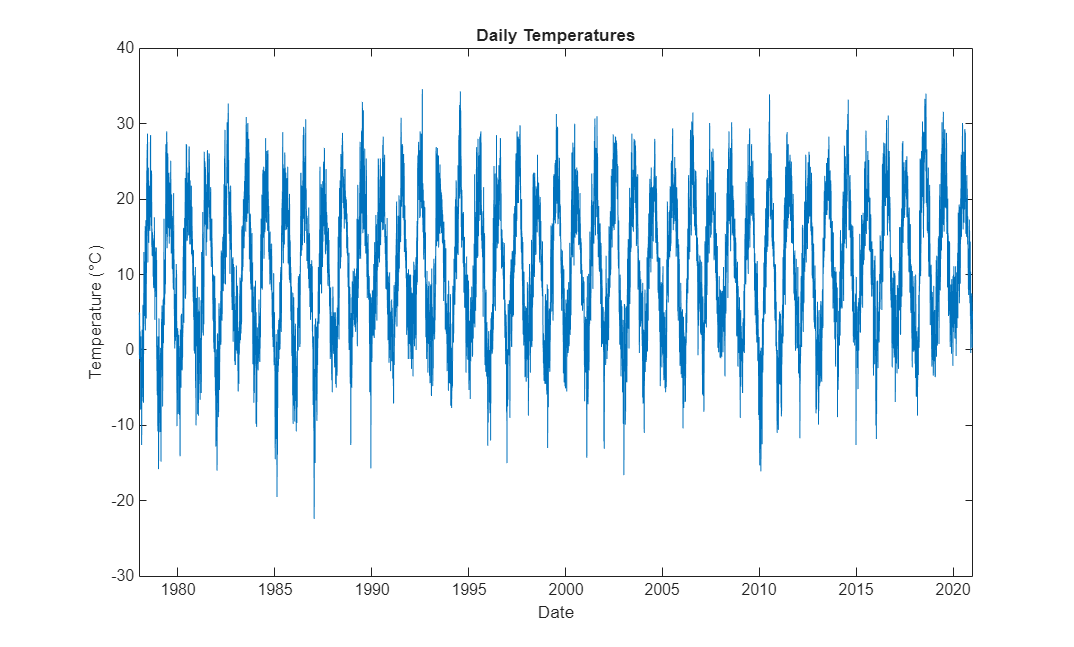In the remaining sections of this example, you model the temperature and then evalauate sample option prices.

### Determine Seasonality in Data

Assume that the deterministic component of the temperature model comprises a linear trend and seasonal terms. To estimate the main frequencies present in the time series, apply the Fourier transform.

Use `determineSeasonality` in Local Functions to remove the linear trend by subtracting the best-fit line. Then, use the `periodogram` (Signal Processing Toolbox) function to compute the spectrum (with a sample rate of one observation per day), and to visualize the spectrum. In addition, the `determineSeasonality` function identifies the top two component frequencies and periods in the data and adds these to the power spectrum.

`determineSeasonality(Temp)`The plot illustrates that the dominant seasonal components in the data are the annual and 6-monthly cycles.

### Fit Deterministic Trend

Based on the previous results, fit the following function for the temperature using the `fitlm` function.

`$\mathrm{TemperatureFcn}=\mathit{A}+\mathit{B}\text{\hspace{0.17em}}\mathit{t}+\mathit{C}\text{\hspace{0.17em}}\mathrm{sin}\left(2\pi \text{\hspace{0.17em}}\mathit{t}\right)+\mathit{D}\text{\hspace{0.17em}}\mathrm{cos}\left(2\pi \text{\hspace{0.17em}}\mathit{t}\right)+\mathit{E}\text{\hspace{0.17em}}\mathrm{cos}\left(4\pi \text{\hspace{0.17em}}\mathit{t}\right)$`

```elapsedTime = years(Temp.Date - Temp.Date(1)); designMatrix = @(t) [t, cos( 2 * pi * t ), sin( 2 * pi * t ), cos( 4 * pi * t )]; trendPreds = ["t", "cos(2*pi*t)", "sin(2*pi*t)", "cos(4*pi*t)"]; trendModel = fitlm(designMatrix(elapsedTime), Temp.TMAX, "VarNames", [trendPreds, "Temperature"])```
```trendModel = Linear regression model: Temperature ~ 1 + t + cos(2*pi*t) + sin(2*pi*t) + cos(4*pi*t) Estimated Coefficients: Estimate SE tStat pValue ________ _________ _______ __________ (Intercept) 9.7095 0.064138 151.39 0 t 0.054648 0.0025836 21.152 6.1629e-98 cos(2*pi*t) -10.909 0.045348 -240.56 0 sin(2*pi*t) -2.772 0.045357 -61.115 0 cos(4*pi*t) 0.39298 0.045348 8.6659 4.9102e-18 Number of observations: 15706, Error degrees of freedom: 15701 Root Mean Squared Error: 4.02 R-squared: 0.798, Adjusted R-Squared: 0.798 F-statistic vs. constant model: 1.55e+04, p-value = 0 ```

Use the `plotDeterministicTrend` in Local Functions to visualize the fitted trend.

`trendModel = plotDeterministicTrend(Temp, trendModel, 1978, 2020)`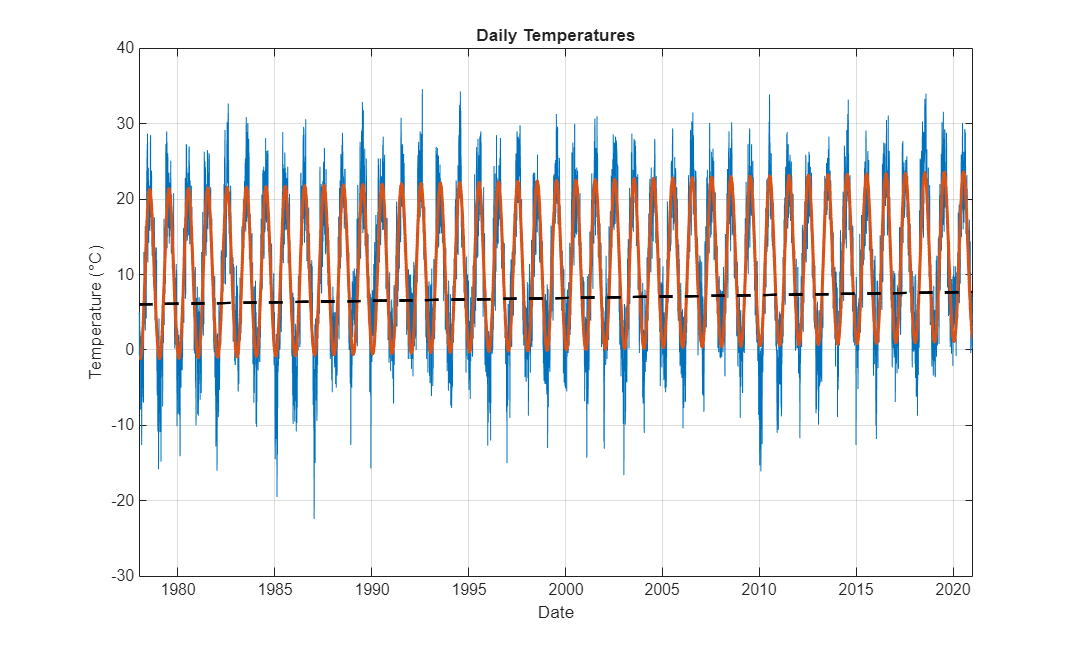```trendModel = Linear regression model: Temperature ~ 1 + t + cos(2*pi*t) + sin(2*pi*t) + cos(4*pi*t) Estimated Coefficients: Estimate SE tStat pValue ________ _________ _______ __________ (Intercept) 9.7095 0.064138 151.39 0 t 0.054648 0.0025836 21.152 6.1629e-98 cos(2*pi*t) -10.909 0.045348 -240.56 0 sin(2*pi*t) -2.772 0.045357 -61.115 0 cos(4*pi*t) 0.39298 0.045348 8.6659 4.9102e-18 Number of observations: 15706, Error degrees of freedom: 15701 Root Mean Squared Error: 4.02 R-squared: 0.798, Adjusted R-Squared: 0.798 F-statistic vs. constant model: 1.55e+04, p-value = 0 ```

The coefficient of the $t$ term is significant with a value of `0.038`. This value suggests that this geographic location has a warming trend of approximately `0.038`°C per year.

### Analyze and Fit Model Residuals

Create an ARIMA/GARCH model for the residuals using `arima` (Econometrics Toolbox).

Based on the preceding results, create a separate time-series model for the regression model residuals using the following workflow:

1. Create an `arima` (Econometrics Toolbox) object and use the `ARMA` terms help to model the autocorrelation in the residual series.

2. Set the constant term to `0`, since the regression model above already includes a constant.

3. Use a $t$-distribution for the innovation series.

4. Use a `garch` (Econometrics Toolbox) model for the variance of the residuals to model the autocorrelation in the squared residuals.

```trendRes = trendModel.Residuals.Raw; resModel = arima( "ARLags", 1, ... "MALags", 1, ... "Constant", 0, ... "Distribution", "t", ... "Variance", garch( 1, 1 ) ); resModel = estimate(resModel,trendRes);```
``` ARIMA(1,0,1) Model (t Distribution): Value StandardError TStatistic PValue ________ _____________ __________ __________ Constant 0 0 NaN NaN AR{1} 0.76851 0.0063873 120.32 0 MA{1} 0.075023 0.010201 7.3545 1.9168e-13 DoF 10.369 0.75567 13.722 7.4771e-43 GARCH(1,1) Conditional Variance Model (t Distribution): Value StandardError TStatistic PValue ________ _____________ __________ __________ Constant 0.67469 0.091761 7.3527 1.9426e-13 GARCH{1} 0.82015 0.019319 42.453 0 ARCH{1} 0.070964 0.0069394 10.226 1.5119e-24 DoF 10.369 0.75567 13.722 7.4771e-43 ```

The residuals are not normally distributed or independent. Also, the residuals show evidence of GARCH effects.

### Simulate Future Temperature Scenarios

Now that you have a calibrated temperature model, you can use it to simulate future temperature paths.

Prepare the simulation time.

```nDays = 730; simDates = Temp.Date(end) + caldays(1:nDays).'; simTime = years(simDates - Temp.Date(1));```

Use `predict` to predict the deterministic trend.

`trendPred = predict(trendModel, designMatrix(simTime));`

Simulate from the ARIMA/GARCH model using `simulate` (Econometrics Toolbox).

`simRes = simulate(resModel, nDays, "NumPaths", 1000, "Y0", trendRes);`

Add the deterministic trend to the simulated residuals.

`simTemp = simRes + trendPred;`

Use `visualizeScenarios` in Local Functions to visualize the temperature scenarios.

`visualizeScenarios(Temp, simTemp, simDates)`### Evaluate Number of Heating Degree Days from Simulation

The number of heating degree days is given by $H=\sum _{i=1}^{n}\text{max}\left(18-{T}_{i},0\right)$, where ${T}_{1},{T}_{2},\dots ,{T}_{n}$ are the simulated temperatures.

```H = sum(max(18 - simTemp, 0)); figure histogram(H) xlabel("HDDs") ylabel("Count") title("Heating Degree Days Distribution") grid on```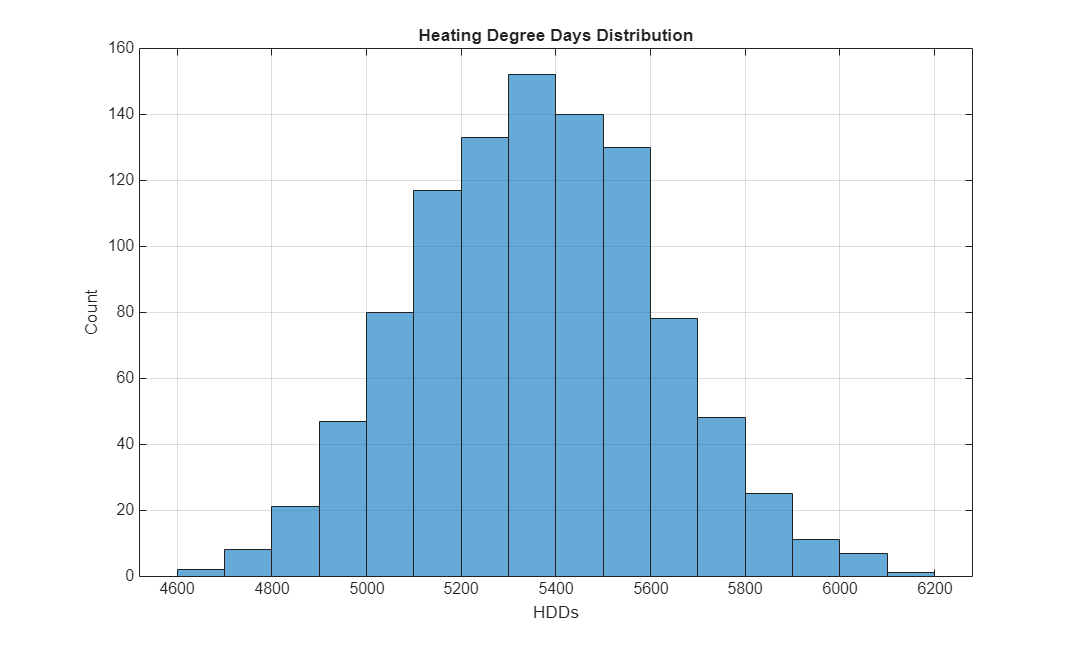Using the original formula $\chi =\alpha \text{\hspace{0.17em}}\mathrm{max}\left\{{\mathit{H}}_{\mathit{n}}-\mathit{K},0\right\}$from Alaton , you can obtain a payoff distribution. When `K` = `7400`, the `max` of `H_n-K` and `0` is always `0` for the set of simulation results in this example. Consequently, the histogram shows the distribution of 1000 zeros, that is, a single bar centered at `0` with a height of `1000`. As `K` decreases to `5000`, `H_n-K` has more opportunities to be positive, so the histogram changes shape.

```K =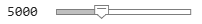5000; figure alpha = 1; chi = alpha*max(H-K, 0); % Payoff of option histogram(chi) title("Option Payoff Distribution")```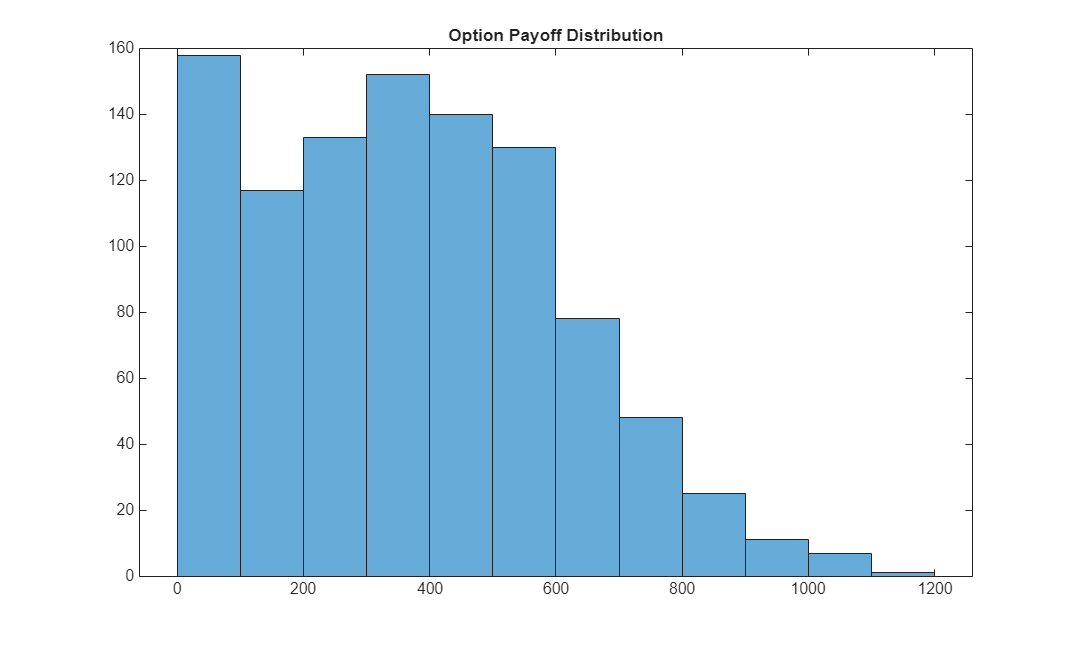### Price Sample Call and Put Options

The weather derivative option pricing calculation is based on `H` (the number of heating degree days) which is defined in the Evaluate Number of Heating Degree Days From Simulation section.

First, compute the mean and standard deviation of H.

```muH = mean(H); sigmaH = std(H);```

Define the sample option parameters.

```r = 0.01; % Risk-free interest rate K = 5000; % Strike value TTM = 0.25; % Expiry time ```

Evaluate α.

`a = (K - muH) / sigmaH;`

Compute the price of the call and put options.

`call_option = exp(-r*TTM) * ((muH - K) * normcdf(-a) + (sigmaH / sqrt(2*pi) * exp(-0.5*a^2)))`
```call_option = 370.1949 ```
`put_option = exp(-r*TTM) * ((K - muH) * (normcdf(a) - normcdf(-muH / sigmaH)) + sigmaH / sqrt(2*pi) * (exp(-0.5*a^2) - exp(-0.5*(muH/sigmaH)^2)))`
```put_option = 8.1038 ```

### References

 Alaton, P., Djehiche, B. and D. Stillberger, D. On Modelling and Pricing Weather Derivatives. Applied Mathematical Finance. 9(1): 1–20, 2002.

 Climate Data Online: Web Services Documentation at https://www.ncdc.noaa.gov/cdo-web/webservices/v2/.

### Local Functions

```function TT_out = getGHCNData(stationID,Element,startDate,endDate,useNOAAinterface) % Function to retrieve GHCN Data % Set flag to use NOAA interface if ~exist("useNOAAinterface","var") || isempty(useNOAAinterface) useNOAAinterface = false; end % For more information on the NOAA interface, see % https://www.mathworks.com/matlabcentral/fileexchange/119213-matlab-interface-for-noaa-data % Get data using noaa interface or readtable from NOAA site if useNOAAinterface n = noaa("WebServicesTokenString"); % Must request data in 1 year increments yearRange = year([startDate;endDate]); TT_Full_From_NOAA = []; for y = yearRange(1):yearRange(2) TT_Full_Year = getdata(n,"GHCND",datetime(y,1,1),datetime(y,12,31),stationid = strcat("GHCND:",stationID),datatypeid = Element,limit = "1000"); TT_Full_From_NOAA = vertcat(TT_Full_From_NOAA,TT_Full_Year); %#ok Date = datetime(TT_Full_From_NOAA.date,"InputFormat",'uuuu-MM-dd''T''HH:mm:SS'); TT_out = timetable(Date,TT_Full_From_NOAA.value/10,'VariableNames',{Element}); end else % Import options for GHCN Data files. For more information, see: % https://www1.ncdc.noaa.gov/pub/data/ghcn/daily/readme.txt DataStartLine = 1; NumVariables = 31*4 + 4; varNames_Array = (["Value","MFlag","QFlag","SFlag"] + (1:31)')'; VariableNames = ["ID","Year","Month","Element",varNames_Array(:)']; VariableWidths = [11 4 2 4 repmat([5 1 1 1],1,31)]; DataType = [{'char','double','double','char'} ... repmat({'double' 'char' 'char' 'char'},1,31)]; opts = fixedWidthImportOptions('NumVariables',NumVariables, ... 'DataLines',DataStartLine, ... 'VariableNames',VariableNames, ... 'VariableWidths',VariableWidths, ... 'VariableTypes',DataType); % Read the full data set. TT_Full = readtable("https://www1.ncdc.noaa.gov/pub/data/ghcn/daily/all/" + ... stationID + ".dly",opts); % Extract the Element data. TT_Element_Full = TT_Full(TT_Full.Element == string(Element),["ID" "Year" ... "Month" "Element" "Value" + (1:31)]); reqYears = year(startDate):year(endDate); TT_Element = TT_Element_Full(ismember(TT_Element_Full.Year,reqYears),:); fullyearDays = datetime(year(startDate),1,1):datetime(year(endDate),12,31); tmpData = TT_Element{:,5:end}'; tmpData = tmpData(:); tmpData(tmpData == -9999) = []; % Remove any empty data Date = (startDate:endDate)'; reqIndex = ismember(fullyearDays,Date); TT_out = timetable(Date,tmpData(reqIndex)/10,'VariableNames',{Element}); end end function determineSeasonality(Temp) % Assume that the deterministic component of the temperature model comprises a linear trend and seasonal terms. % To estimate the main frequencies present in the time series, apply the Fourier transform. % First, remove the linear trend by subtracting the best-fit line. detrendedTemps = detrend(Temp.TMAX); % Next, use the periodogram function to compute the spectrum. The sampling frequency is one observation per day. numObs = length(detrendedTemps); Fs = 1; [pow, freq] = periodogram(detrendedTemps, [], numObs, Fs); % Visualize the spectrum. powdB = db(pow); figure plot(freq, powdB) xlabel("Frequency (days^{-1})") ylabel("Power (dB)") title("Detrended Temperatures Power Spectrum") grid on % Identify the top two component frequencies and periods in the data. [topPow, idx] = findpeaks(powdB, "NPeaks", 2, ... "SortStr", "descend", ... "MinPeakProminence", 20); topFreq = freq(idx); topPeriods = 1 ./ topFreq; % Add the top two component frequencies and periods to the spectrum. hold on plot(topFreq, topPow, "r^", "MarkerFaceColor", "r") xlim([min( topFreq ) - 1e-3, max( topFreq ) + 1e-3]) legend("Spectrum", "Top periods (days): " + join( string( topPeriods ), ", " )) % Note that the dominant seasonal components in the data are the annual and 6-monthly cycles. end function trendModel = plotDeterministicTrend(Temp, trendModel, from, to) % Visualize the fitted trend. figure; plot(Temp.Date, Temp.TMAX) xlabel("Date") ylabel("Temperature (" + char( 176 ) + "C)") title("Daily Temperatures") grid on hold on plot(Temp.Date, trendModel.Fitted, "LineWidth", 2) plot(Temp.Date,6.06+0.038*years(Temp.Date - Temp.Date(1)),'k--','LineWidth',2) xlim([datetime(from,1,1), datetime(to,12,31)]) end function visualizeScenarios(Temp, simTemp, simDates) figure plot(Temp.Date, Temp.TMAX) hold on plot(simDates, simTemp) % Plot the simulation percentiles. simPrc = prctile(simTemp, [2.5, 50, 97.5], 2); plot(simDates, simPrc, "y", "LineWidth", 1.5) xlim([Temp.Date(end) - calyears(1), simDates(end)]) xlabel("Date") ylabel("Temperature (" + char( 176 ) + "C)") title("Temperature Simulation") grid on % Get the data for 2021. stationID = 'SW000008525'; Element = 'TMAX'; startDate = datetime(2021,1,1); endDate = datetime(2021,12,31); T = getGHCNData(stationID,Element,startDate,endDate); plot(T.Date, T.TMAX, "g", "LineWidth", 1.5) end```